Скачать презентацию Model checking 1 And now

8356cfaa8475fa89a55d119d647e3fd6.ppt

• Количество слайдов: 27Model checking 1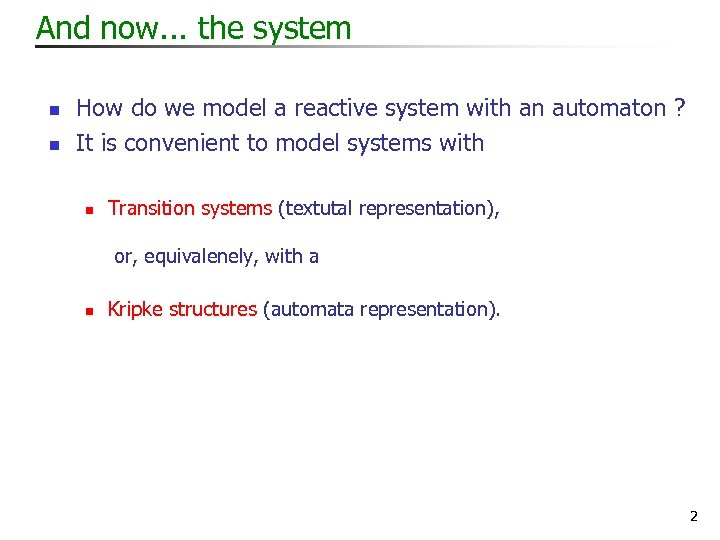And now. . . the system n n How do we model a reactive system with an automaton ? It is convenient to model systems with n Transition systems (textutal representation), or, equivalenely, with a n Kripke structures (automata representation). 2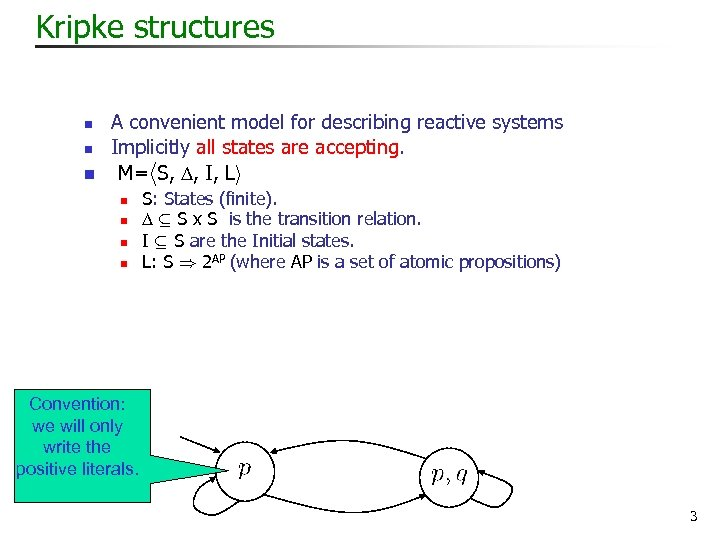Kripke structures n n n A convenient model for describing reactive systems Implicitly all states are accepting. M=h. S, , I, Li n n S: States (finite). µ S x S is the transition relation. I µ S are the Initial states. L: S ) 2 AP (where AP is a set of atomic propositions) Convention: we will only write the positive literals. 3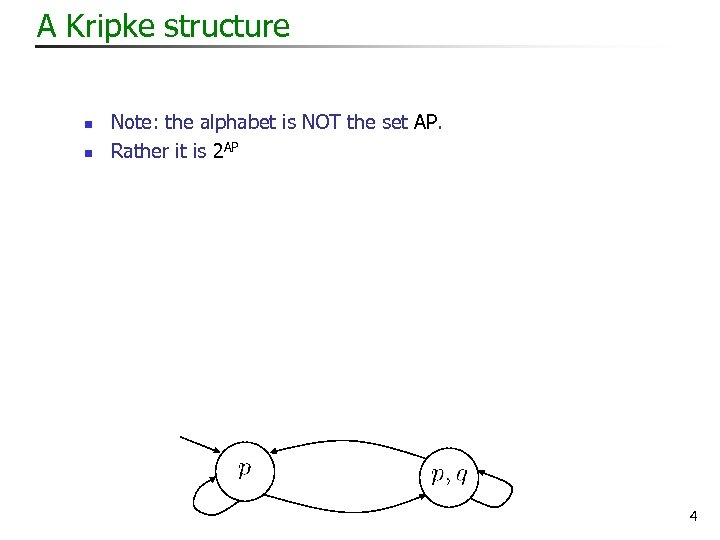A Kripke structure n n Note: the alphabet is NOT the set AP. Rather it is 2 AP 4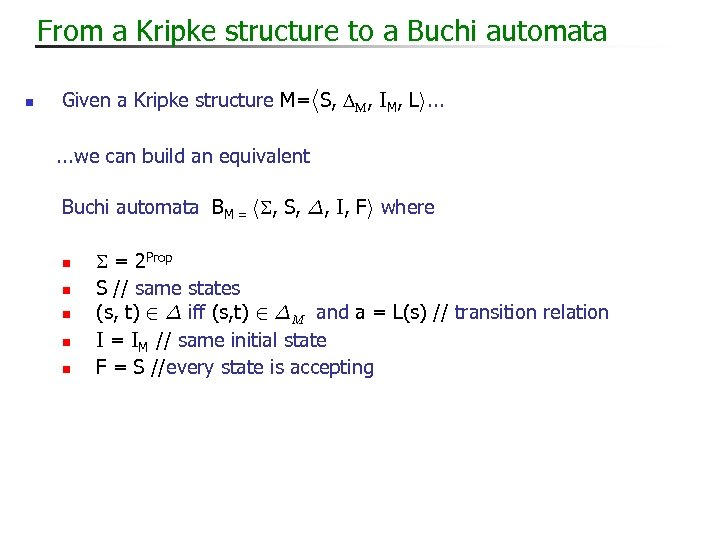From a Kripke structure to a Buchi automata n Given a Kripke structure M=h. S, M, IM, Li. . . we can build an equivalent Buchi automata BM = h , S, ¢, I, Fi where n n n = 2 Prop S // same states (s, t) 2 ¢ iff (s, t) 2 ¢M and a = L(s) // transition relation I = IM // same initial state F = S //every state is accepting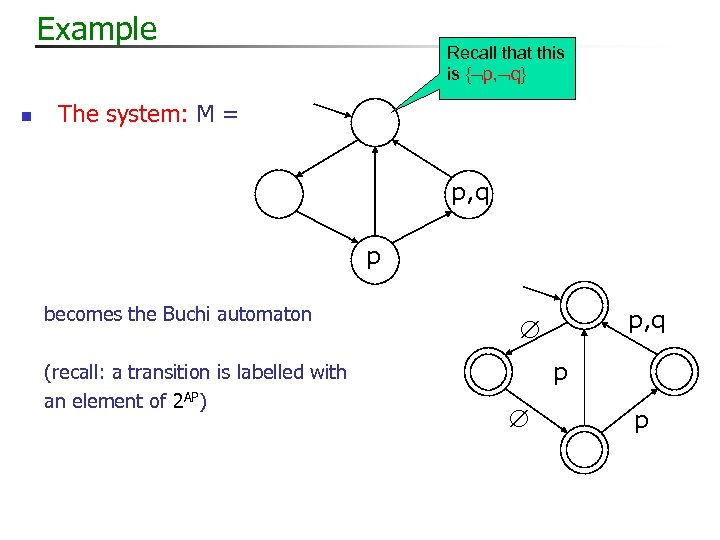Example n Recall that this is { p, q} The system: M = p, q p becomes the Buchi automaton (recall: a transition is labelled with an element of 2 AP) p, q p p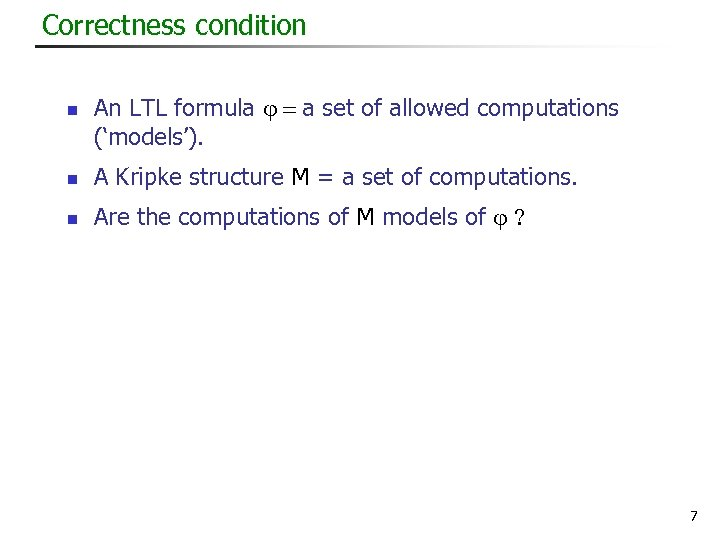Correctness condition n An LTL formula = a set of allowed computations (‘models’). n A Kripke structure M = a set of computations. n Are the computations of M models of ? 7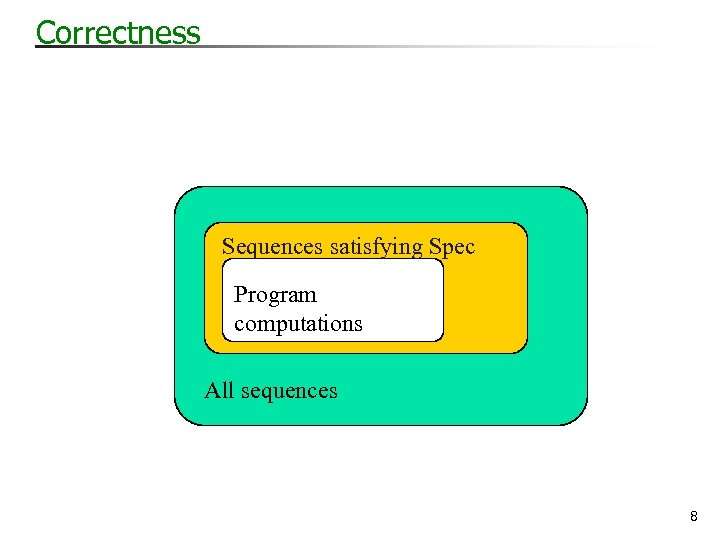Correctness Sequences satisfying Spec Program computations All sequences 8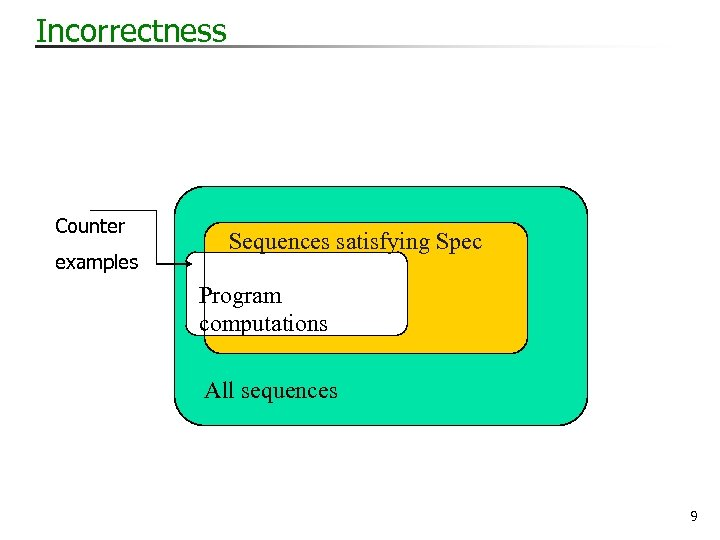Incorrectness Counter examples Sequences satisfying Spec Program computations All sequences 9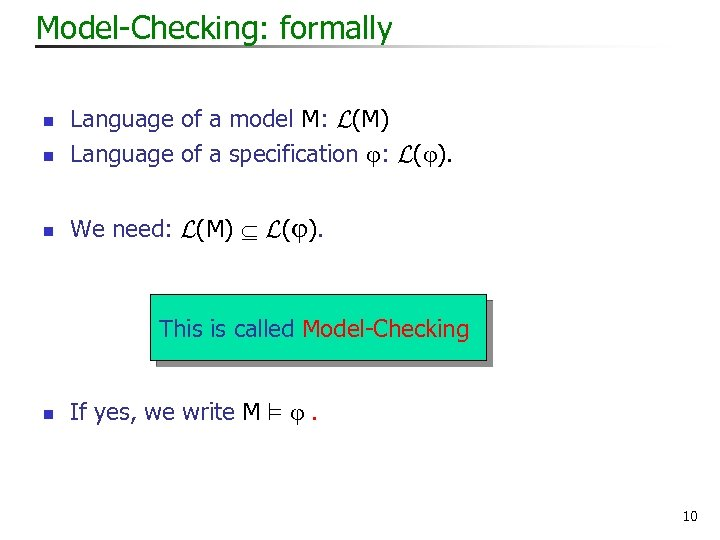Model-Checking: formally n Language of a model M: L(M) Language of a specification : L( ). n We need: L(M) L( ). n This is called Model-Checking n If yes, we write M ² . 10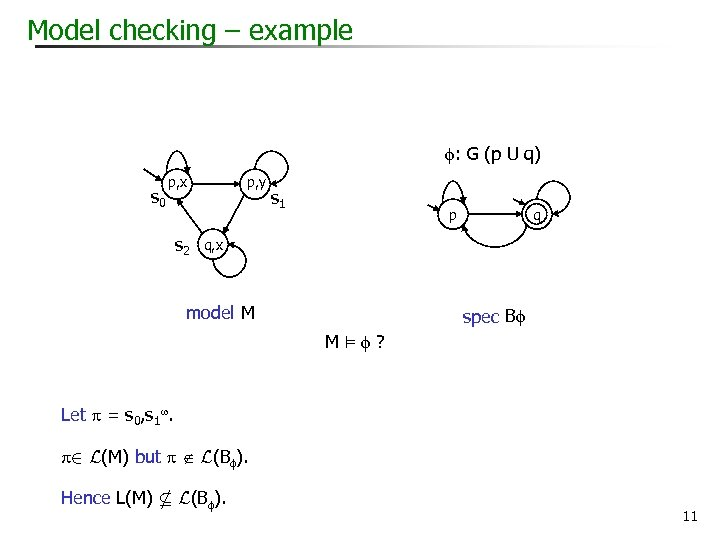Model checking – example : G (p U q) s 0 p, y p, x s 2 s 1 p q q, x model M spec B M² ? Let = s 0, s 1. 2 L(M) but L(B ). Hence L(M) * L(B ). 11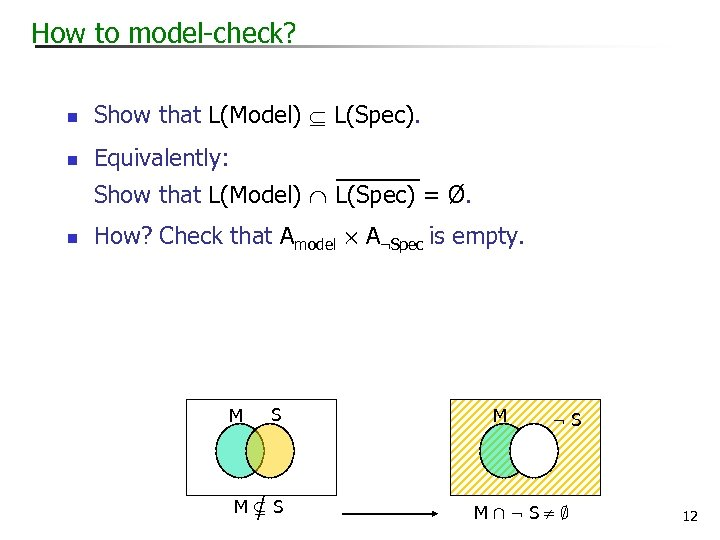How to model-check? n Show that L(Model) L(Spec). n Equivalently: Show that L(Model) L(Spec) = Ø. n How? Check that Amodel £ A: Spec is empty. M S MµS M : S MÅ: S¹; 12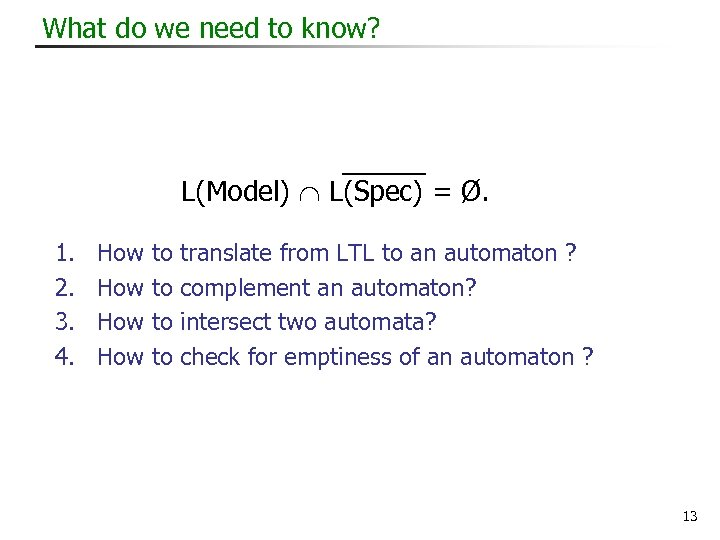What do we need to know? L(Model) L(Spec) = Ø. 1. 2. 3. 4. How How to to translate from LTL to an automaton ? complement an automaton? intersect two automata? check for emptiness of an automaton ? 13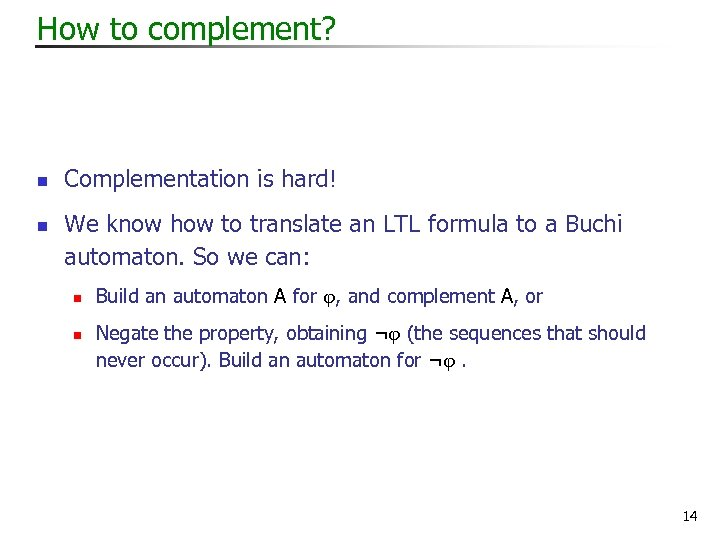How to complement? n n Complementation is hard! We know how to translate an LTL formula to a Buchi automaton. So we can: n n Build an automaton A for , and complement A, or Negate the property, obtaining ¬ (the sequences that should never occur). Build an automaton for ¬ . 14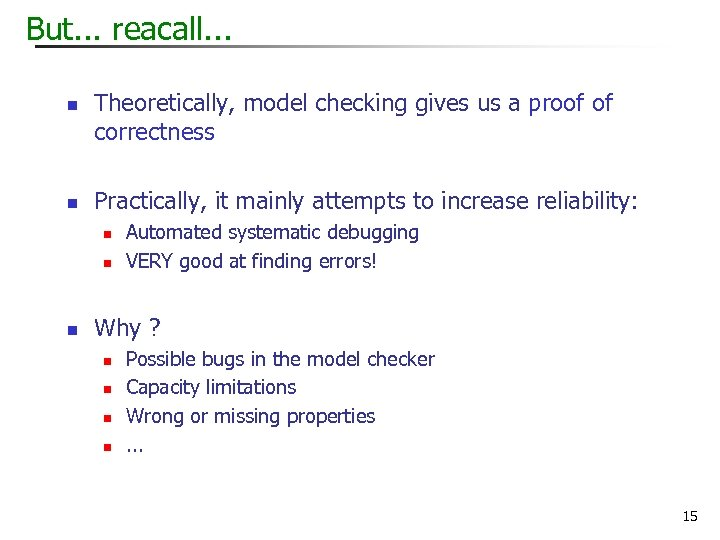But. . . reacall. . . n n Theoretically, model checking gives us a proof of correctness Practically, it mainly attempts to increase reliability: n n n Automated systematic debugging VERY good at finding errors! Why ? n n Possible bugs in the model checker Capacity limitations Wrong or missing properties. . . 15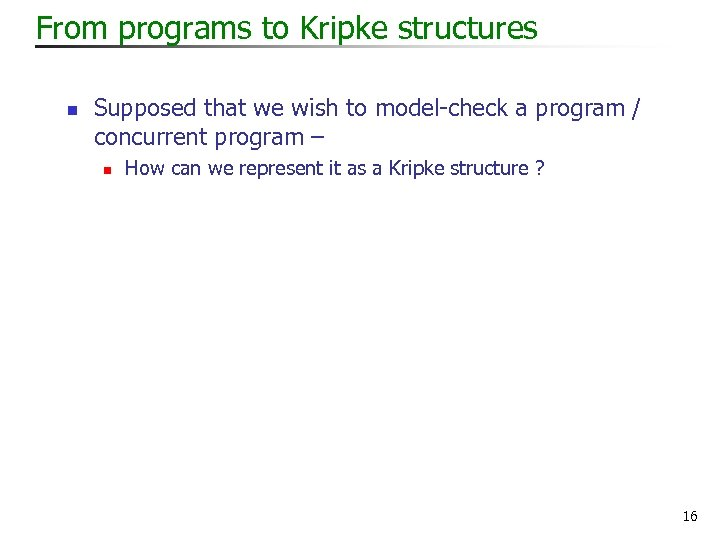From programs to Kripke structures n Supposed that we wish to model-check a program / concurrent program – n How can we represent it as a Kripke structure ? 16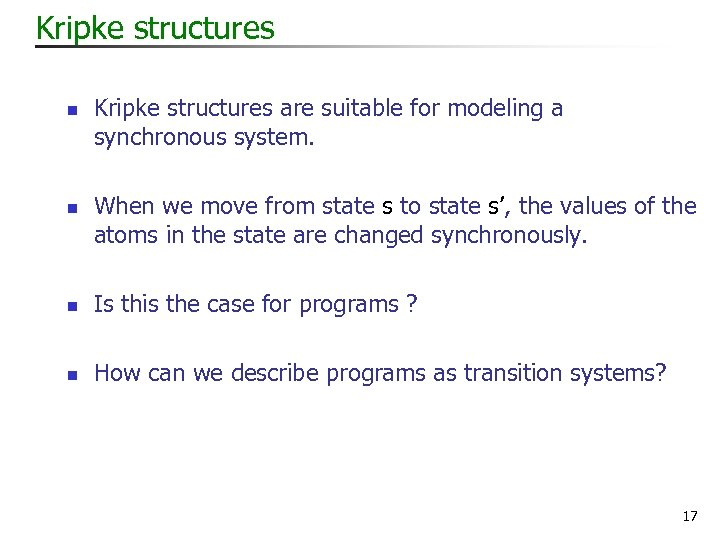Kripke structures n n Kripke structures are suitable for modeling a synchronous system. When we move from state s to state s’, the values of the atoms in the state are changed synchronously. n Is this the case for programs ? n How can we describe programs as transition systems? 17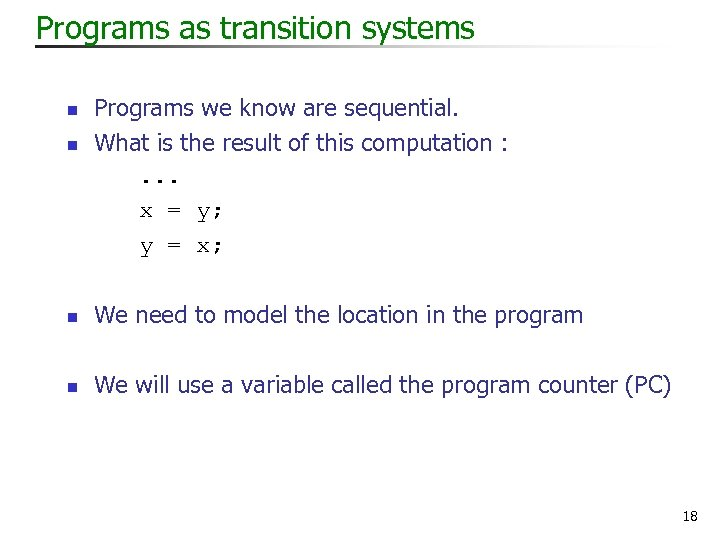Programs as transition systems n n Programs we know are sequential. What is the result of this computation : . . . x = y; y = x; n We need to model the location in the program n We will use a variable called the program counter (PC) 18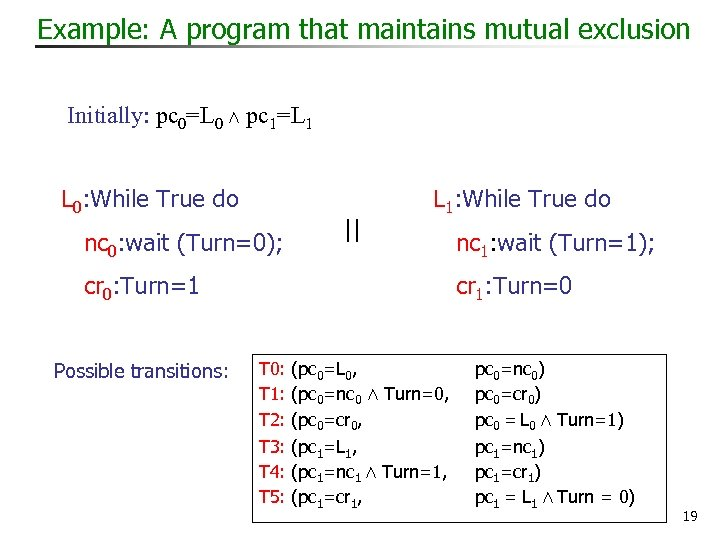Example: A program that maintains mutual exclusion Initially: pc 0=L 0 Æ pc 1=L 1 L 0: While True do nc 0: wait (Turn=0); || L 1: While True do cr 0: Turn=1 Possible transitions: nc 1: wait (Turn=1); cr 1: Turn=0 T 0: (pc 0=L 0, T 1: (pc 0=nc 0 Æ Turn=0, T 2: (pc 0=cr 0, pc 0=nc 0) pc 0=cr 0) pc 0 = L 0 Æ Turn=1) T 3: (pc 1=L 1, T 4: (pc 1=nc 1 Æ Turn=1, T 5: (pc 1=cr 1, pc 1=nc 1) pc 1=cr 1) pc 1 = L 1 Æ Turn = 0) 19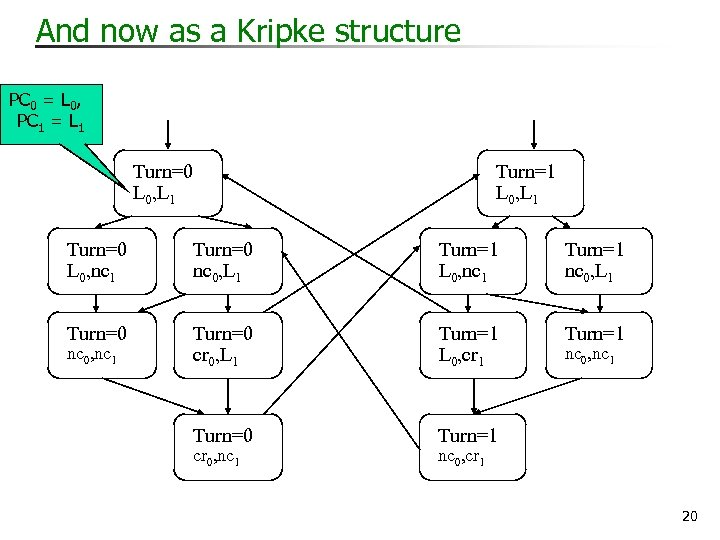And now as a Kripke structure PC 0 = L 0, PC 1 = L 1 Turn=0 L 0, L 1 Turn=1 L 0, L 1 Turn=0 L 0, nc 1 Turn=0 nc 0, L 1 Turn=1 L 0, nc 1 Turn=1 nc 0, L 1 Turn=0 cr 0, L 1 Turn=1 L 0, cr 1 Turn=0 Turn=1 nc 0, nc 1 cr 0, nc 1 nc 0, cr 1 20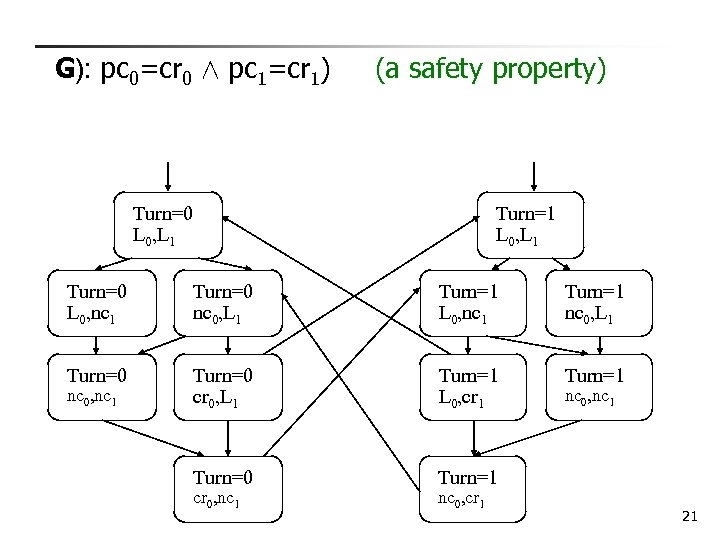G): pc 0=cr 0 Æ pc 1=cr 1) (a safety property) Turn=0 L 0, L 1 Turn=1 L 0, L 1 Turn=0 L 0, nc 1 Turn=0 nc 0, L 1 Turn=1 L 0, nc 1 Turn=1 nc 0, L 1 Turn=0 cr 0, L 1 Turn=1 L 0, cr 1 Turn=0 Turn=1 nc 0, nc 1 cr 0, nc 1 nc 0, cr 1 nc 0, nc 1 21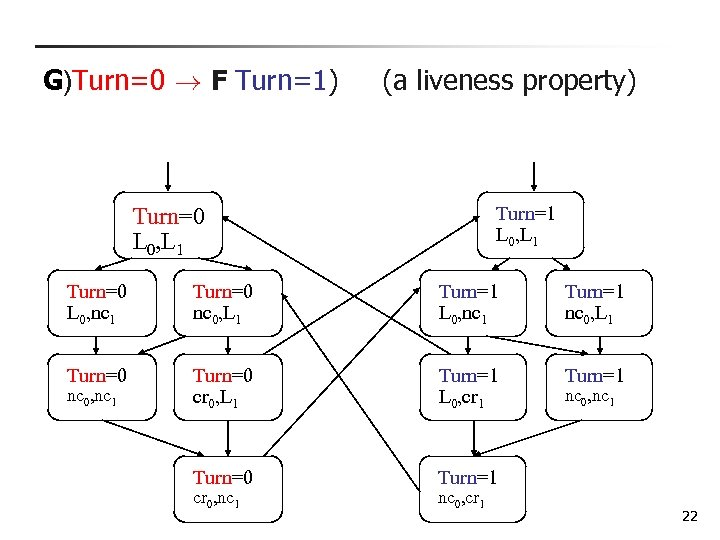G)Turn=0 ! F Turn=1) (a liveness property) Turn=1 L 0, L 1 Turn=0 L 0, nc 1 Turn=0 nc 0, L 1 Turn=1 L 0, nc 1 Turn=1 nc 0, L 1 Turn=0 cr 0, L 1 Turn=1 L 0, cr 1 Turn=0 Turn=1 nc 0, nc 1 cr 0, nc 1 nc 0, cr 1 nc 0, nc 1 22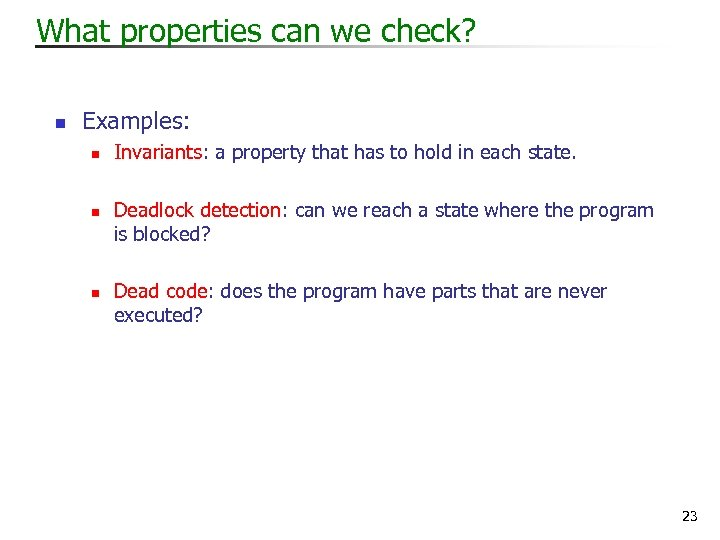What properties can we check? n Examples: n n n Invariants: a property that has to hold in each state. Deadlock detection: can we reach a state where the program is blocked? Dead code: does the program have parts that are never executed? 23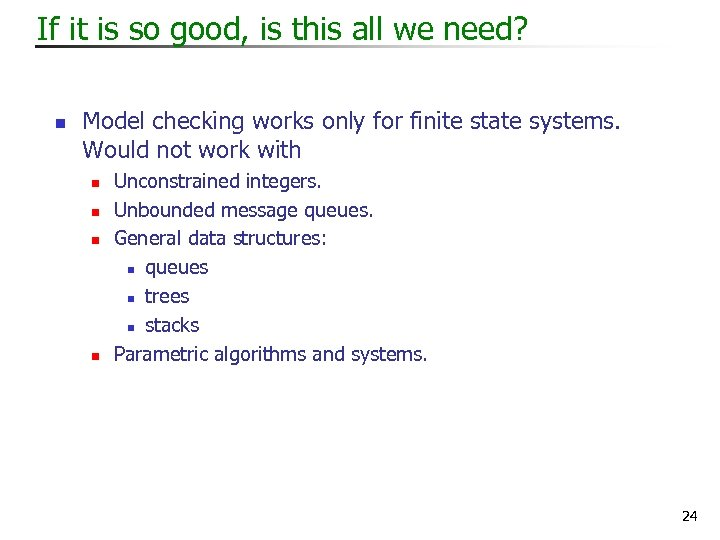If it is so good, is this all we need? n Model checking works only for finite state systems. Would not work with n n Unconstrained integers. Unbounded message queues. General data structures: n queues n trees n stacks Parametric algorithms and systems. 24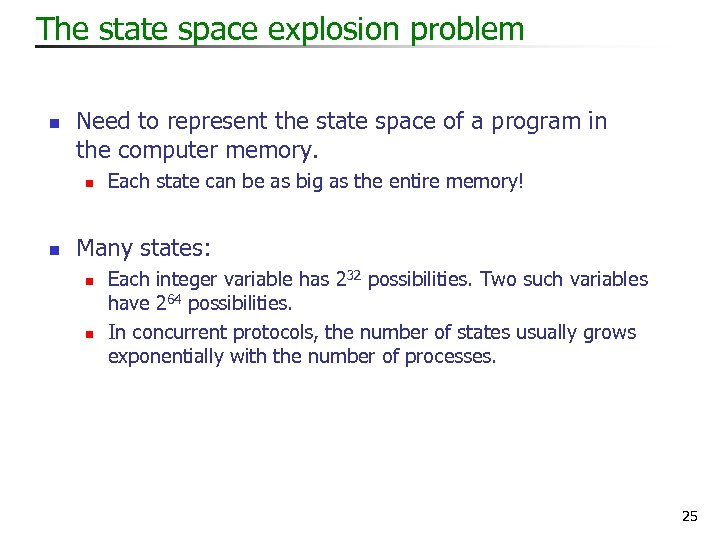The state space explosion problem n Need to represent the state space of a program in the computer memory. n n Each state can be as big as the entire memory! Many states: n n Each integer variable has 232 possibilities. Two such variables have 264 possibilities. In concurrent protocols, the number of states usually grows exponentially with the number of processes. 25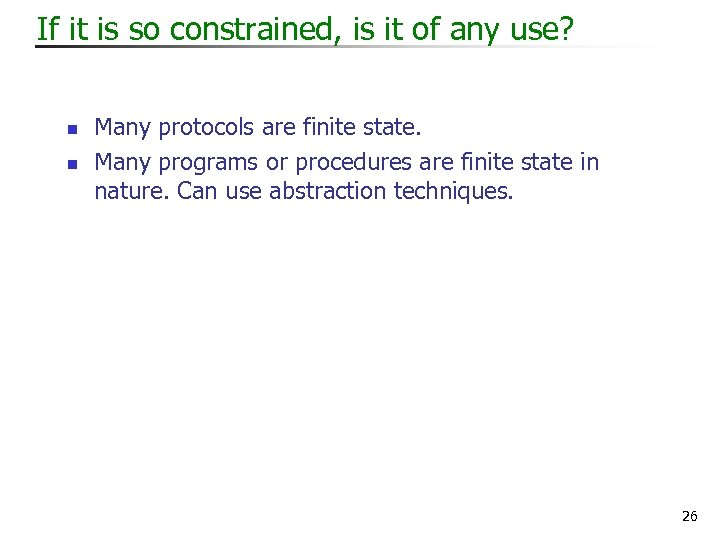If it is so constrained, is it of any use? n n Many protocols are finite state. Many programs or procedures are finite state in nature. Can use abstraction techniques. 26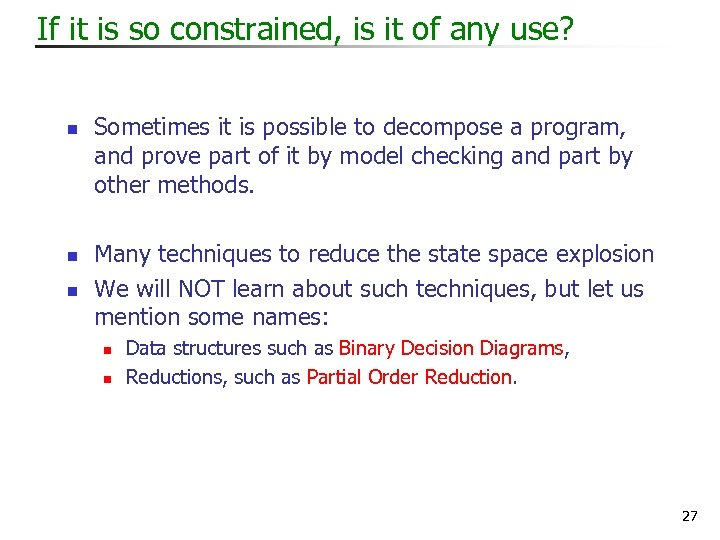If it is so constrained, is it of any use? n n n Sometimes it is possible to decompose a program, and prove part of it by model checking and part by other methods. Many techniques to reduce the state space explosion We will NOT learn about such techniques, but let us mention some names: n n Data structures such as Binary Decision Diagrams, Reductions, such as Partial Order Reduction. 27